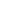# Free CBSE Class 8 Maths Worksheets

Download free printable Maths Worksheets to practice. With thousands of questions available, you can generate as many Maths Worksheets as you want.

## Sample CBSE Class 8 Maths Worksheet Questions

1.

There are two boys, Aman and Sumit. Aman's age is two times Sumit's age. Then if present age of Aman is 2 years what will be the age of Sumit after 50 years?

1.

25

2.

49

3.

50

4.

51

2.

pqr is what type of polynomial?

1.
Monomial

2.

Binomial

3.

Trinomial

4.

None of these

3.

1.  Which of the following is the numerical coefficient of x2y2?

1.

0

2.

1

3.

x2

4.

y2

4.

What is the area of the recatangle with the width of 6 and length of 12 ?

1.

18

2.

72

3.

72

4.

18

5.

Rational numbers are numbers that:-

1.

Can be written as fraction

2.

3.

Are only posotive

4.

Never repeat and never end.

6.

Which of the following types of number is not a rational?

1.

Fraction.

2.

Mixed number.

3.

pi.

4.

repeating decimal.

7.

Simplify. (2x + 5)2 – (2x – 5)2

1.

20h

2.

40h

3.

30h

4.

60h

8.

Use the identity (h + a) (h + b) = h2 + (a + b) h + ab to find product. 501 × 502

1.

251502

2.

251501

3.

9.

2197 is perfect cube root of

1.

12

2.

13

3.

14

4.

15

10.

10648 is perfect cube root of

1.

22

2.

23

3.

24

4.

28

## Find more Maths Worksheets

Worksheets by UrbanPro

Our worksheets are designed to help students explore various topics, practice skills and enrich their subject knowledge, to improve their academic performance. Designed by Experts who have extensive experience and expertise in teaching a subject, these worksheets will improve your child's problem-solving skills and subject knowledge in a fun and interactive manner.
Check out our free customized worksheets across school boards, grades, subjects and levels of subject knowledge. You can download, print and share these worksheets with anyone, anywhere, anytime!

Get a custom worksheet to practice!

Select your topic & see the magic.

subjectSelect Chapter(s)

Chapters & Subtopics